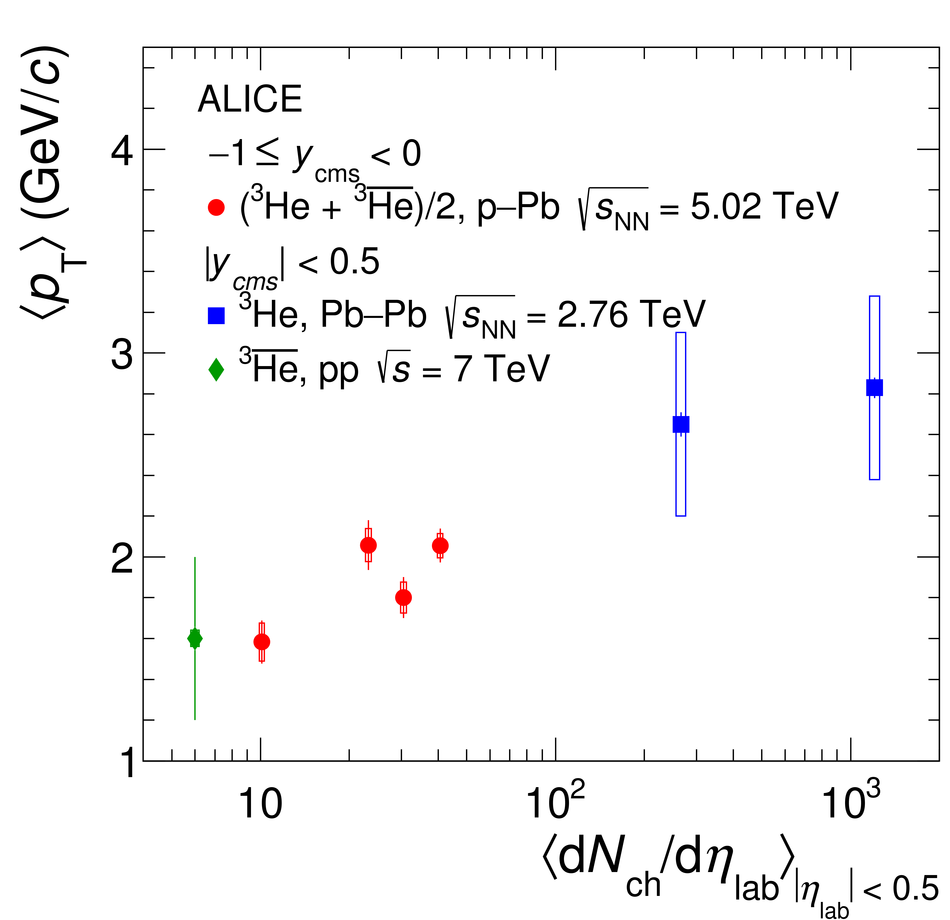# Figure 6

 Left: Mean transverse momentum of (anti-)$^3$He as a function of the mean charged-particle multiplicity density in p--Pb collisions at $\energy = 5.02$ TeV. Statistical and systematic uncertainties are indicated by vertical bars and boxes, respectively. The published results from pp and Pb--Pb collisions are shown with diamonds and rectangles, respectively Right: Mean transverse momentum measured in p--Pb collisions at $\energy = 5.02$ TeV as a function of the particle mass is shown for different mean charged-particle multiplicity densities. The linear scaling with the mass found for the results for $\pi$, K, p, $\Lambda$ , $\Xi$, and $\Omega$ is indicated by dashed lines. The deuteron $\langle p_{\mathrm{T}}\rangle$ is taken from .